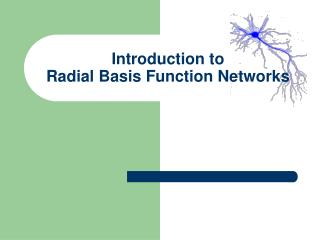DownloadDownload PresentationIntroduction to Radial Basis Function Networks

# Introduction to Radial Basis Function Networks

Télécharger la présentation## Introduction to Radial Basis Function Networks

- - - - - - - - - - - - - - - - - - - - - - - - - - - E N D - - - - - - - - - - - - - - - - - - - - - - - - - - -
##### Presentation Transcript

1. Introduction to Radial Basis Function Networks

2. Content • Overview • The Models of Function Approximator • The Radial Basis Function Networks • RBFN’s for Function Approximation • The Projection Matrix • Learning the Kernels • Bias-Variance Dilemma • The Effective Number of Parameters • Model Selection • Incremental Operations

3. Typical Applications of NN • Pattern Classification • Function Approximation • Time-Series Forecasting

4. f ˆ f Function Approximation Unknown Approximator

5. + +  Supervised Learning Unknown Function Neural Network

6. Neural Networks as Universal Approximators • Feedforward neural networks with a single hidden layer of sigmoidal units are capable of approximating uniformly any continuous multivariate function, to any desired degree of accuracy. • Hornik, K., Stinchcombe, M., and White, H. (1989). "Multilayer Feedforward Networks are Universal Approximators," Neural Networks, 2(5), 359-366. • Like feedforward neural networks with a single hidden layer of sigmoidal units, it can be shown that RBF networks are universal approximators. • Park, J. and Sandberg, I. W. (1991). "Universal Approximation Using Radial-Basis-Function Networks," Neural Computation, 3(2), 246-257. • Park, J. and Sandberg, I. W. (1993). "Approximation and Radial-Basis-Function Networks," Neural Computation, 5(2), 305-316.

7. Statistics vs. Neural Networks

8. Introduction to Radial Basis Function Networks The Model of Function Approximator

9. Linear Models Weights Fixed Basis Functions

10. y w2 w1 wm 1 2 m x1 x2 xn x = Linear Models Linearly weighted output Output Units • Decomposition • Feature Extraction • Transformation Hidden Units Inputs Feature Vectors

11. Linear Models Can you say some bases? y Linearly weighted output Output Units w2 w1 wm • Decomposition • Feature Extraction • Transformation Hidden Units 1 2 m Inputs Feature Vectors x1 x2 xn x =

12. Example Linear Models Are they orthogonal bases? • Polynomial • Fourier Series

13. y w2 w1 wm 1 2 m x1 x2 xn x = Single-Layer Perceptrons as Universal Aproximators With sufficient number of sigmoidal units, it can be a universal approximator. Hidden Units

14. y w2 w1 wm 1 2 m x1 x2 xn x = Radial Basis Function Networks as Universal Aproximators With sufficient number of radial-basis-function units, it can also be a universal approximator. Hidden Units

15. Non-Linear Models Weights Adjusted by the Learning process

16. Introduction to Radial Basis Function Networks The Radial Basis Function Networks

17. Radial Basis Functions • Center • Distance Measure • Shape Three parameters for a radial function: i(x)= (||x  xi||) xi r = ||x  xi|| 

18. Typical Radial Functions • Gaussian • Hardy Multiquadratic • Inverse Multiquadratic

19. Gaussian Basis Function (=0.5,1.0,1.5)

20. Inverse Multiquadratic c=5 c=4 c=3 c=2 c=1

21. + + + Basis {i: i =1,2,…} is `near’ orthogonal. Most General RBF

22. Properties of RBF’s • On-Center, Off Surround • Analogies with localized receptive fields found in several biological structures, e.g., • visual cortex; • ganglion cells

23. y1 ym x1 x2 xn As a function approximator The Topology of RBF Output Units Interpolation Hidden Units Projection Inputs Feature Vectors

24. y1 ym x1 x2 xn As a pattern classifier. The Topology of RBF Output Units Classes Hidden Units Subclasses Inputs Feature Vectors

25. Introduction to Radial Basis Function Networks RBFN’s for Function Approximation

26. Unknown Function to Approximate Training Data The idea y x

27. Unknown Function to Approximate Training Data Basis Functions (Kernels) The idea y x

28. Function Learned Basis Functions (Kernels) The idea y x

29. Nontraining Sample Function Learned Basis Functions (Kernels) The idea y x

30. Nontraining Sample Function Learned The idea y x

31. w2 w1 wm x1 x2 xn x = Radial Basis Function Networks as Universal Aproximators Training set Goal for all k

32. w2 w1 wm x1 x2 xn x = Learn the Optimal Weight Vector Training set Goal for all k

33. Regularization Training set If regularization is unneeded, set Goal for all k

34. Learn the Optimal Weight Vector Minimize

35. Learn the Optimal Weight Vector

36. Learn the Optimal Weight Vector Design Matrix Variance Matrix

37. Training set Summary

38. Introduction to Radial Basis Function Networks The Projection Matrix

39. Unknown Function The Empirical-Error Vector

40. Unknown Function The Empirical-Error Vector Error Vector

41. If =0, the RBFN’s learning algorithm is to minimizeSSE (MSE). Sum-Squared-Error Error Vector

42. The Projection Matrix Error Vector

43. Introduction to Radial Basis Function Networks Learning the Kernels

44. y1 yl wlml wl1 wl2 w1m w11 w12 2 m 1 x1 x2 xn RBFN’s as Universal Approximators Training set Kernels

45. y1 yl wlml wl1 wl2 w1m w11 w12 2 m 1 x1 x2 xn What to Learn? • Weightswij’s • Centers j’s of j’s • Widthsj’s of j’s • Number of j’s  Model Selection

46. One-Stage Learning

47. The simultaneous updates of all three sets of parameters may be suitable for non-stationary environments or on-line setting. One-Stage Learning